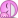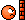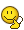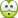# Sad mistakes from students

Gold Member
I’m a student and, of course, and I make a whole lot of too many mistakes, but while occasionally helping my fellow peers, I’ve seen really--it hurts to say it--awful mistakes that shouldn’t even be mistakes. Like, a 16-year-old not knowing how to use FOIL, or being unable to graph a parabola or circle. One time, I even saw this: ##(5\log)x=5(\log x)## … what does that even mean?? What makes it worse is that it’s not coming from students who plan to just get out of school as soon as possible--these mistakes come from people saying they want to go to a good university and pursue all these highly credited careers. I find it really sad. So I was wondering, for all you teachers/professors/academics … is that really how it is everywhere? Or am I just stuck with a “special” batch of people? If the former, do you just get frustrated and upset and let the student know? Do you ignore the mistakes and just not care?

Being a teacher of a higher level class only to discover that those you are teaching can't even do basic work sounds extremely disheartening.

## Answers and Replies

fresh_42
Mentor
I’m a student and, of course, and I make a whole lot of too many mistakes, but while occasionally helping my fellow peers, I’ve seen really--it hurts to say it--awful mistakes that shouldn’t even be mistakes. Like, a 16-year-old not knowing how to use FOIL, or being unable to graph a parabola or circle. One time, I even saw this: ##(5\log)x=5(\log x)## … what does that even mean?? What makes it worse is that it’s not coming from students who plan to just get out of school as soon as possible--these mistakes come from people saying they want to go to a good university and pursue all these highly credited careers. I find it really sad. So I was wondering, for all you teachers/professors/academics … is that really how it is everywhere? Or am I just stuck with a “special” batch of people? If the former, do you just get frustrated and upset and let the student know? Do you ignore the mistakes and just not care?

Being a teacher of a higher level class only to discover that those you are teaching can't even do basic work sounds extremely disheartening.
The very first phrase of our constitution is: The dignity of man is untouchable. (We have a gender neutral word for man, though.)
One of my favorite bumper stickers I ever saw was: The stupidity of man is untouchable.

It's a truth and as you see right now on every news channel: stupidity doesn't prevent people from getting famous, successful or rich.
The first year at our universities is usually the toughest one because the difference to school is quite big. There has been a favorite path for students: signed up in macroeconomic → found too much math → changed to microeconomic → still math, ... → finally registered to study laws.

However, you may not forget that even good students have bad days or just make mistakes on carelessness or due to time pressure. There are some mentors here I really admire for their patience with my mistakes or those of others. I guess one has to get more experienced than a 16 year old can be to handle it with calm. For now: get a laugh at (by yourself, not to others). This way they are at least good for something.

Last edited by a moderator:
•gracy
Gold Member
There has been a favorite path for students: signed up in macroeconomic → found too much math → changed to microeconomic → still math ... → finally registered to study laws.
That's a comic(in a ... really sad way ...)
However, you may not forget that even good students have bad days or just make mistakes on carelessness or due to time pressure.
Oh, of course, I’m not denying that! Just the other day I found myself plotting a graph of lab data with the independent variable on the y-axis … and then I started analyzing the daft-looking data and completing the report (explaining all the possible errors affecting the conclusion) without the slightest idea on where I went wrong.
For now: get a laugh at (by yourself, not to others). This way they are at least good for something.
*sigh* I don’t find funny. Forgetting how to find the slope of a line in 11th grade is not funny. Sure, I can playfully tease and you get a laugh from the others, but it’s still sadLast edited by a moderator:
Once I taught a refresher course in math for prospective science students. I stressed a million times that ##(x+y)^2 = x^2 + 2xy + y^2##. So they really got that.

So when the time of the test came, sure enough, a lot of them solved ##(x+2)^2 = x^2 + 4x + 4## correctly. I was happy. But then most of them also said ##(x+y)^3= x^3 + y^3##. SighGold Member
So when the time of the test came, sure enough, a lot of them solved ##(x+2)2=x2+4x+4(x+2)^2 = x^2 + 4x + 4## correctly. I was happy. But then most of them also said ##(x+y)3=x3+y3(x+y)^3= x^3 + y^3##. SighDo you find it depressing or do you simply ignore it? Are you one of those teachers who will yell in front of class telling everyone how disappointed you are or do you smile and make fun of it?

There was once this person who was trying to solve a system of equations and he was supposed to use Gaussian elimination. I told him to make an augmented matrix to derive something in row-echelon form and then … he asked what a matrix was.

Doesn’t something like that bother you?

Astronuc
Staff Emeritus
Science Advisor
So when the time of the test came, sure enough, a lot of them solved (x+2)2=x2+4x+4(x+2)2=x2+4x+4(x+2)^2 = x^2 + 4x + 4 correctly. I was happy. But then most of them also said (x+y)3=x3+y3(x+y)3=x3+y3(x+y)^3= x^3 + y^3. SighPerhaps you meanor perhaps less concussivelyIt didn't occur so some that ##(x+y)^3 = (x+y)^2(x+y)##

Unfortunately, I feel like a lot of this results from people treating math like they treat, say, history. They often think "well, we've had our test on the FOIL method, now I can forget that and learn the next topic."

As an old professor of mine used to say, if you're in a history class and you didn't do well in the 1500s, you can still do well in the 1600s. That's not the case with math, though. Students need to have it stressed that, yes, they do need to remember all this stuff. It's not something you can forget right after the test, because math is cumulative.

A lot of it, I think, occurs because many teachers teach math as a set of arbitrary rules for manipulating formulas into particular forms that look completely uninteresting to the layman. And, don't get me wrong, we do need to have those rules drilled into our heads until we get it right. But oh, how much more beautifully math could be taught.

•ProfuselyQuarky, billy_joule and cnh1995
blue_leaf77
Science Advisor
Homework Helper
During one mathematical physics class, a friend of mine was asked by the lecturer to go in front of the class to work out certain differentiation problem $$\frac{d\,f(x)}{dx}$$, I don't remember the particular form of ##f(x)##. Then she nonchalantly stroke out the ##d##'s in the numerator and denominator as if it acts like a multiplication, and ended up with a meme-type equation
$$\frac{d\,f(x)}{dx} = \frac{f(x)}{x}$$
Strangely though, she demonstrated that unintended joke in front of the class but only a (very) few of us noticed that that was laughable. The majority of the class seemed to either agree with her work or didn't know themself what to be done with that problem. I thought if she had been given other non-trivial mathematical expressions and was asked to simplify it, she might set up a new trend of math meme.
Such people are basically lacking in the information needed to properly tackle the problem they are given, at the same time they use "intuition" based on what they already have learned - a misuse of concept.

•ProfuselyQuarky and cnh1995
I took a University math prep class last September - equivalent to grade 12 mathematics, essentially precalculus. During our trigonometry unit test, one of my classmates was solving sin(14° + 46°). This is trivial to any who has taken trigonometry and was meant to be a gimme, but unfortunately one of my classmates took the degree symbol to be a zero exponent, and sin(2) was her answer..She nearly cried when informed of her mistake a few days later.

•gracy
I took a University math prep class last September - equivalent to grade 12 mathematics, essentially precalculus. During our trigonometry unit test, one of my classmates was solving sin(14° + 46°). This is trivial to any who has taken trigonometry and was meant to be a gimme, but unfortunately one of my classmates took the degree symbol to be a zero exponent, and sin(2) was her answer..She nearly cried when informed of her mistake a few days later.

To be fair, degrees are stupid.

•member 587159
cnh1995
Homework Helper
Gold Member
I don't remember the particular form of f(x)f(x)f(x). Then she nonchalantly stroke out the ddd's in the numerator and denominator as if it acts like a multiplication, and ended up with a meme-type equation
My maths professor once carelessly stroke out variables and messed up the entire solution. While simplifying an expression, he stroke out the x's in log(x)/(x) and wrote it as log(x)/x=log(1)=0. Coincidently, the expected final answer was also 0, so he didn't realize his mistake until one of us brought it to his notice. He still does such things. He's famous among the students for doing such silly things and has earned a nickname too!

Some student actually turned this in on an exam, and expected partial credit because he had the right answer:

$$\int_0^{2\pi}\cos x dx = \left.\frac{\sin x}{x}\right|_0^{2\pi} = \frac{\sin(2\pi)}{2\pi} - \frac{\sin(0)}{0} = \sin - \sin = 0$$

From: http://www.math.vanderbilt.edu/~schectex/commerrs/

•collinsmark
collinsmark
Homework Helper
Gold Member
Some student actually turned this in on an exam, and expected partial credit because he had the right answer:

$$\int_0^{2\pi}\cos x dx = \left.\frac{\sin x}{x}\right|_0^{2\pi} = \frac{\sin(2\pi)}{2\pi} - \frac{\sin(0)}{0} = \sin - \sin = 0$$
What in the..... That's just <loss for words>. I don't even know where to begin.

fresh_42
Mentor
$$\int_0^{2\pi}\cos x dx = \left.\frac{\sin x}{x}\right|_0^{2\pi} = \frac{\sin(2\pi)}{2\pi} - \frac{\sin(0)}{0} = \sin - \sin = 0$$
I can't help but admire the artistic creativity. I love modern art.
I mean, NASA once crashed a Mars probe because Lockheed and them used different systems of unities ...

•Drakkith and ProfuselyQuarky
Reminds me of the old $$\require{cancel} \frac{64}{16} = \frac{\cancel{6}4}{1\cancel{6}} = \frac{4}{1} = 4$$ That's why the process is just as important as the answer!

•ProfuselyQuarky and gracy
fresh_42
Mentor
Reminds me of the old $$\require{cancel} \frac{64}{16} = \frac{\cancel{6}4}{1\cancel{6}} = \frac{4}{1} = 4$$ That's why the process is just as important as the answer!
... or why multiplication points not always should be omitted, resp. conventions about notation has to be clearly defined.

Gold Member
Reminds me of the old $$\require{cancel} \frac{64}{16} = \frac{\cancel{6}4}{1\cancel{6}} = \frac{4}{1} = 4$$ That's why the process is just as important as the answer!
It's hard to believe that that is a real mistake some one made ...
To be fair, degrees are stupid.
To be fair, the use of radians in daily measurements is stupid.

It's hard to believe that that is a real mistake some one made ...

I don't know that anyone's ever actually done that (though you never know...) but it's more of an example of how just because a method gives the correct result does not mean that method will always work.

•ProfuselyQuarky
fresh_42
Mentor
It's hard to believe that that is a real mistake someone made ...
Why? ##\require{cancel} \frac{64}{16} = \frac{6 \cdot 4}{1 \cdot 6} = \frac{\cancel{6} \cdot 4}{1 \cdot \cancel{6}} = \frac{4}{1} = 4##•cnh1995 and ProfuselyQuarky
To be fair, the use of radians in daily measurements is stupid.

Burn the heretic!

•fresh_42
Gold Member
Why? ##\require{cancel} \frac{64}{16} = \frac{6 \cdot 4}{1 \cdot 6} = \frac{\cancel{6} \cdot 4}{1 \cdot \cancel{6}} = \frac{4}{1} = 4##Haha. Let's see ...

##\frac {63}{9}=\frac {6\cdot3}{3\cdot3}=\frac {\cancel{3}\cdot\cancel{3}\cdot2}{\cancel {3}\cdot\cancel{3}}=2##

Ah, accuracy.
Burn the heretic!
I'm willing to die a martyr for what I believeLast edited:
fresh_42
Mentor
Burn the heretic!
Exactly. Don't mess with Leonhard!

fresh_42
Mentor
Haha. Let's see ...

##\frac {63}{9}=\frac {6\cdot3}{3\cdot3}=\frac {\cancel{3}\cdot\cancel{3}\cdot2}{\cancel {3}\cdot\cancel{3}}=2##

Ah, accuracy.
##\frac{63}{9} ≡ \frac{3}{4} ≡ 3 \cdot 4 ≡ 12 ≡ 2 \mod 5 ## - Only a matter of perspective•ProfuselyQuarky
vela
Staff Emeritus
Science Advisor
Homework Helper
Education Advisor
Some student actually turned this in on an exam, and expected partial credit because he had the right answer:

$$\int_0^{2\pi}\cos x dx = \left.\frac{\sin x}{x}\right|_0^{2\pi} = \frac{\sin(2\pi)}{2\pi} - \frac{\sin(0)}{0} = \sin - \sin = 0$$

From: http://www.math.vanderbilt.edu/~schectex/commerrs/
I can believe the part of the student wanting partial credit. I find it hard to believe anyone would actually turn that in! How did they end up in a calculus class?

DrGreg
Science Advisor
Gold Member
This wasn't a "sad mistake" but a deliberate abuse of notation (as a joke) from my maths teacher when I was 15.

To solve: $$\frac{dy}{dx} = y$$Integrate:$$y = \int y + C$$Rearrange:$$y - \int y = C$$Factorise:$$\left(1 - \int \right) y = C$$Divide:$$y = \frac{C}{1 - \int}$$Expand the geometric series:\begin{align*} y &= C + \int C + \int^2 C + \int^3 C + \dots \\ &= C + \int C + \iint C + \iiint C + \dots \\ &= C + Cx + \frac{Cx^2}{2} + \frac{Cx^3}{3!} + \dots \\ &= Ce^x \end{align*}which is the correct answer.

Of course the method is nonsense for a 15-year old, but years later when I studied functional analysis and operator theory, I realised the method is essentially correct (if you rewrite it with more appropriate symbols, terminology and apply the correct terms and conditions). I don't know if my maths teacher had known this or not.

•cnh1995, ProfuselyQuarky, fresh_42 and 2 others